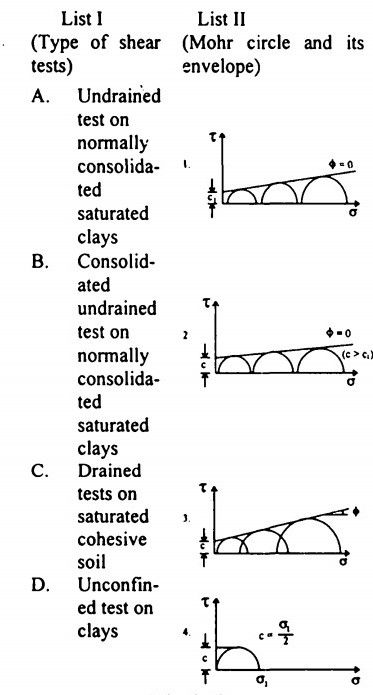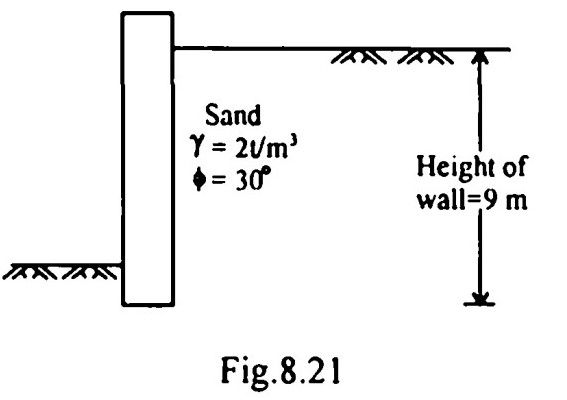top of pageSearch

# Soil Mechanics (321-340)

Updated: Apr 28, 2020

321. If the saturated density of a given soil is 2.1 t/m², then the total stress (T in t/m²)

and the effective stress (E in t/m²) of a saturated soil stratum at a depth of 4 m

T=8.4 E=4.4

322. The void ratio-pressure diagram is shown in Fig.8.17.The coefficient of compressibility is

0.20 m²/t

323. Bearing capacity of a soil strata supporting a footing of size 3 m x 3 m will

not be affected by the presence of ground water table located at a depth which is

3 m below the base of the footing

324. Consider the following statements regarding negative skin friction in piles:

1. It is developed when the pile is driven through a recently deposited clay layer.

2. It is developed due to a sudden draw down of the water table

325. A concentrated load of 50 t acts vertically x at a point on the soil surface. If

Boussinesq's equation is applied for computation of stress, then the ratio of

vertical stresses at depths of 3 m and 5 m respectively vertically below the point of

application of load will be 2.77

326. Efficiency of a pile group is defined as327. No tension should develop at the base of the rectangular well foundation or at any horizontal section within the well. For no tension at the base, the resultant of 'Pa' (Total active thrust) and 'W (Weight of soil and well above the base) must pass through middle third of the base

328.

Modulus of sub-grade reaction-Plate load test Relative density and strength-Standard penetration test Skin friction and point bearing-Cyclic pile load test Elastic constant-Pressure meter test

329.A soil sample is having a specific gravity of 2.60 and a void ratio of 0.78. The water content in percentage required to fully saturate the soil at that void ratio would be 30

330. A bed of sand consists of three horizontal layers of equal thickness. The value of Darcy's k for the upper and lower layers is 1 x 10⁻² cm/sec and that for the middle layer is 1 x lO⁻¹cm/sec. The ratio of the permeability of the bed in the horizontal direction to that in the vertical direction is 2.8 to 1

331. A soil has liquid limit of 60%, plastic limit of 35% and shrinkage limit of 20% and it has a natural moisture content of 50%. The liquidity index of soil is 0.6

332.Sheep-foot rollers are recommended for compacting cohesive soils

333.A deposit of fine sand has a porosity n and specific gravity of soil solids is G. The hydraulic gradient of the deposit to develop boiling condition of sand is given

by334.In the schematic flow-net shown in Fig.8.18, the hydraulic potential at point

A is12 m of water

335. Consider the following statements in relation to the given sketch:1.Soil is partially saturated at degree of saturation = 60%

2.Water content = 30%

3.Saturated unit weight = 1.5 g/cc.

336. Which of the following laboratory triaxial test parameters should one specify to be carried out in connection with the initial stability of footing on saturated clay? C, <Φᵤ—Undrained

337. Which one of the following Fig.8.19 gives the failure envelope for a normally

consolidated saturated clay sample tested in triaxial test under drained conditions?338.339. A soil fails under an axial vertical stress of 100 kN/m² in unconfined compression test. The failure plane makes an angle of 50° with the horizontal. The shear parameters c and Φ respectively will be

50.0 kN/m²,10

340.Active earth pressure per metre length on the retaining wall with a smooth vertical back as shown in Fig.8.21 will be.27 t